# Fitting to specific curves

QtiPlot includes quick access to the most useful functions for fitting.

## Fitting to a line

This command is used to fit a curve which has a linear shape.

Figure 6-4. The results of a Fit Linear (Default).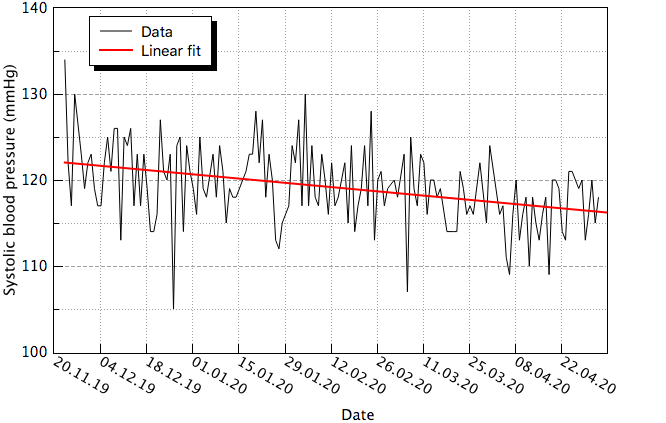The results will be given in the Log panel:## Fitting to a polynomial

This command is used to fit a polynomial function to data which has a curvilinear shape. It opens the Polynomial Fit Options dialog, allowing you to choose the curve to fit, the order of the polynomial function to use, the number of points of the resulting curve and the abscissa limits for the fit.

The results of the fit are displayed in the Log panel

Figure 6-5. The results of a Fit Polynomial..., showing the initial data, the curve added to the plot, and the results in the log panel.## Fitting to a Boltzmann function

This command is used to fit a curve which has a sigmoidal shape. The function used is:

Equation 6-3. Boltzmann equationin which A1 is the low Y limit, A2 is the high Y limit, x0 is the inflexion (half amplitude) point and dx is the width.

Figure 6-6. The results of a Fit Boltzmann (sigmoidal).When the X axis is using a logarithmic scale, the Fit Boltzmann (sigmoidal) command uses the Logistical equation for fitting:

Equation 6-4. Logistic dose response equation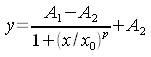where A1 is the initial Y value, A2 is the final Y value, x0 is the inflexion point (center) and p is the power.

## Fitting to a Gauss function

This command is used to fit a curve which has a bell shape. The function used is:

Equation 6-5. Gauss equation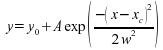in which A is the height, w is the width, xc is the center and y0 is the Y-values offset.

Figure 6-7. The results of a Fit Gaussian.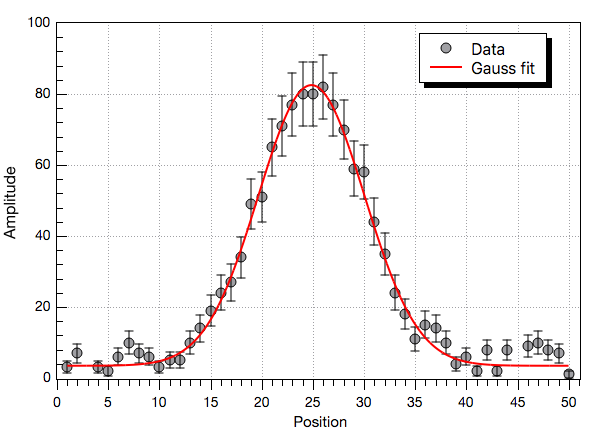## Fitting to a Lorentz function

This command is used to fit a curve which has a bell shape. The function used is:

Equation 6-6. Lorentz equation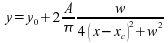in which A is the area, w is the width, xc is the center and y0 is the Y-values offset.

Figure 6-8. The results of a Fit Lorentzian.## Fitting to a PsdVoigt1 function

This command is used to fit a curve with a Pseudo-Voigt function which is a linear combination of Gaussian and Lorentzian functions:

Equation 6-7. PsdVoigt1 equationThe parameters of the PsdVoigt1 function have the following meaning: y0 is the Y-values offset, A is the area, w is the width (FWHM), xc is the center and mu is a profile shape factor.

## Fitting to a PsdVoigt2 function

This command is used to fit a curve with a Pseudo-Voigt function which is a linear combination of Gaussian and Lorentzian functions with different FWHM:

Equation 6-8. PsdVoigt2 equation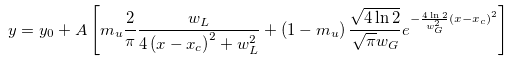The parameters of the PsdVoigt2 function have the following meaning: y0 is the Y-values offset, A is the area, wG is the Gaussian FWHM, wL is the Lorentzian FWHM, xc is the center and mu is a profile shape factor.